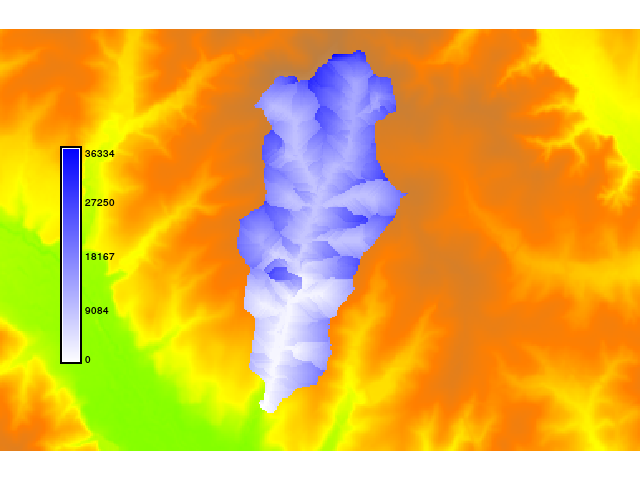## NAME

r.traveltime - Estimation of travel times/isochrones.
Computes the travel time of surface runoff to an outlet

## KEYWORDS

raster, hydrology

## SYNOPSIS

r.traveltime
r.traveltime --help
r.traveltime [-q] dir=name accu=name dtm=name manningsn=name out_x=string out_y=string threshold=string b=string nchannel=string dis=string [slopemin=string] out=name [--overwrite] [--help] [--verbose] [--quiet] [--ui]

### Flags:

-q
Quiet
--overwrite
Allow output files to overwrite existing files
--help
Print usage summary
--verbose
Verbose module output
--quiet
Quiet module output
--ui
Force launching GUI dialog

### Parameters:

dir=name [required]
Flow direction map (e.g. derived by r.watershed)
accu=name [required]
Flow accumulation map (e.g. derived by r.watershed)
dtm=name [required]
Depressionless, filled terrain model (e.g. derived by r.fill.dir)
manningsn=name [required]
Map with Manning's n value for surface roughness
out_x=string [required]
x coordinate of basin outlet
out_y=string [required]
y coordinate of basin outlet
threshold=string [required]
Minimum number of cells (threshold) that classify cell as channel
b=string [required]
Channel width
nchannel=string [required]
Channel roughness (Manning's n)
dis=string [required]
Specific discharge [l/s/km**2]
slopemin=string
Minimum slope for flat areas [m/m]
out=name [required]
Output travel time map [seconds]

## DESCRIPTION

r.traveltime computes the travel time of surface runoff to an outlet. The program starts at the basin outlet and calculates the travel time for each raster cell recursively. A drainage area related threshold considers either surface runoff or channel runoff. Travel times are derived by assuming kinematic wave approximation.
In order to derive channel flow velocities, an equilibrium discharge for each cell is calculated (Q=Area*specific discharge).
The results can be used to derive a time-area function. This might be useful for precipitation-runoff calculations (estimation of flood predictions) with a lumped hydrological model (user-specified unit hydrograph).

## REMARKS

The program ist restricted to SI units (meters). The algorithm is recursive. Maybe it will not work with extensive datasets. It is assumed that the minimum slope is 0.001. For smaller gradients the program uses this value.
Please not that the flow accumulation map must be defined as single direction. Multiple flow directions are not supported. Thus, the "SFD (D8) flow" option has to be set if, e.g., the r.watershed module is used to generate the input files (parameter s). The flow accumulation map should include positive values only (-a of r.watershed). Flow direction definitions are in accordance to the r.fill.dir program using the "agnps" format option.

## KNOWN ISSUES

The program does not work correctly if Manning's roughness grid is defined as double (float expected). To define a simple uniform roughness distribution try: r.mapcalc 'roughness = 0.1f'

## EXAMPLE

This example uses the North Carolina sample dataset.

```g.region raster=elevation
r.mapcalc "n = 0.1f"
r.fill.dir input=elevation output=fill direction=flowdir format=agnps
r.fill.dir input=fill output=fill2 direction=flowdir2 format=agnps
r.watershed -a -s elevation=fill2 accumulation=accu
r.traveltime --overwrite dir=flowdir2 accu=accu dtm=fill2 manningsn=n \
out_x=634613 out_y=217014 threshold=250 b=3 nchannel=0.03 slopemin=0.01 \
dis=900 out=ttime
r.colors ttime colors=blues
```r.watershed, r.fill.dir
http://jesbergwetter.twoday.net/stories/4845555/

## REFERENCES

• Kilgore, J. L. (1997): Development and evaluation of a GIS-based spatially distributed unit hydrograph model, master thesis, Virginia Polytechnic Institute and State University.
• Melesse, A. M., Graham, W. D. (2004): Storm runoff predicition based on a spatially distributed travel time method utilizing remote sensing and GIS, Journal of the American Water Resources Association, 8, 863-879.
• Muzik, I. (1996): Flood modelling with GIS-derived distributed unit hydrographs, Hydrological Processes, 10, 1401-1409.

## AUTHOR

Kristian Foerster

## SOURCE CODE

Available at: r.traveltime source code (history)

Latest change: Monday Jan 30 19:52:26 2023 in commit: cac8d9d848299297977d1315b7e90cc3f7698730

© 2003-2023 GRASS Development Team, GRASS GIS 8.3.1dev Reference Manual